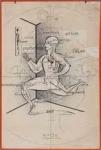### Science Quiz

Random Science or Chemistry Quiz

# Chemistry Laws Named After Scientists Quiz Stats

#### byorgoman  Plays Quiz Updated Jan 29, 2020

Score 0/25 Timer 05:00
5 Plays Today
Description of Law Name of Law % Correct
PV = constant for a given amount of a gas at a constant temperatureBoyle's Law
71.4%
V1/T1 = V2/T2Charles' Law
46.8%
E = hνPlanck relation
46.3%
At the same temperature and pressure, equal volumes of different gasses contain the same number of particlesAvogadro's law
43.9%
No two electrons in a single atom can have the same set of four quantum numbersPauli Exclusion Principle
37.3%
∆x • ∆(pv) ≥ h/Heisenberg Uncertainty Principle
35.6%
ĤΨ = EΨSchrödinger equation
33.1%
Ptot = Pa + Pb + …..Dalton's Law of Partial Pressures
31.6%
Electrons in degenerate orbitals enter singly with parallel spinsHund's Rule
25.2%
k = Ae( –Ea/RT)Arrhenius Equation
25%
If a change is imposed on a system at equilibrium, the position of the equilibrium will shift in a direction that tends to reduce that changeLe Chatelier's principle
24.8%
∆H = ∆H1 + ∆H2 + ……Hess' Law
24.4%
A = ε l c Beer's Law
22.4%
Description of Law Name of Law % Correct
E = (2.31 x 10-19 J nm)(Q1Q2/r)Coulomb's law
22.1%
P/T = constant for a given amount of a gas at a constant volumeGay-Lussac's Law
21.5%
The weight of any element liberated during electrolysis is proportional to the quantity of electricity passing through the cell and also to the equivalent weight of the element. Faraday's Law
20.9%
λ = h/pde Broglie relation
19.5%
The amount of a gas dissolved in a solution is directly proportional to the pressure of the gas above the solutionHenry's Law
19%
pH = pKa + log [A-]/[HA]Henderson-Hasselbalch equation
18.9%
[P + a(n/V)2](V-nb) = nRTvan der Waals Equation
15.5%
ε = εstandard - (RT/nF)ln QNernst Equation
13.7%
Psolvent in solution = Χsolvent PsolventRaoult's Law
13.4%
The relative rates of effusion of two gasses are equal to the inverse of the square roots of thier massesGraham's law of effusion
13.3%
vo = vmax[S]/(KM+[S])Michaelis-Menten equation
6.9%
ln (K2/K1) = ∆Hrxn/R (1/T1-1/T2)Van't Hoff equation
6%

## From the Vault See Another

Blind Secret Country VII

by goc3

Geography 90s
Blindfolded driving is never recommended.